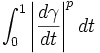Generalized energy functional

Fix a Riemannian manifold. The generalized energy functional is a map from the space of piecewise smooth curves in the manifold, to reals, defined as follows: the generalized energy functional evaluated at a curve$\gamma$ is:$\int_0^1 \left|\frac{d\gamma}{dt}\right|^p dt$
For$p=1$, we get the arc-length functional and for$p=2$, we get the energy functional.